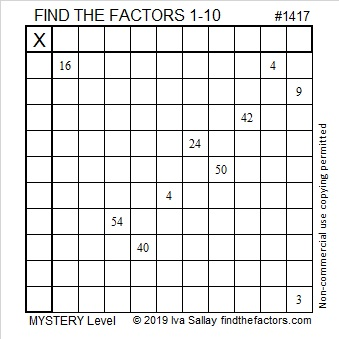# 1417 Mystery Puzzle

How hard is today’s puzzle? It’s a little harder just because I’m not telling what the level number is. Are you going to let that stop you from finding the unique solution? I hope not!Print the puzzles or type the solution in this excel file: 10 Factors 1410-1418

1417 is just the puzzle number, but in case you want to know something about it, here are some facts:

• 1415 is a composite number.
• Prime factorization: 1415 = 13 × 109
• 1415 has no exponents greater than 1 in its prime factorization, so √1415 cannot be simplified.
• The exponents in the prime factorization are 1, and 1. Adding one to each exponent and multiplying we get (1 + 1)(1 + 1) = 2 × 2 = 4. Therefore 1415 has exactly 4 factors.
• The factors of 1415 are outlined with their factor pair partners in the graphic below.1417 is the sum of two squares in two different ways:
29² + 24² = 1417
36² + 11² = 1417

1417 is the hypotenuse of FOUR Pythagorean triples:
265-1392-1417 calculated from 29² – 24², 2(29)(24), 29² + 24²
545-1308-1417 which is (5-12-13) times 109
780-1183-1417 which is 13 times (60-91-109)
792-1175-1417 calculated from 2(36)(11) , 36² – 11² , 36² + 11²

This site uses Akismet to reduce spam. Learn how your comment data is processed.